# RD Sharma Solutions for Class 8 Maths Chapter 3 - Squares and Square Roots Exercise 3.2

Students can refer and download the RD Sharma Solutions for the Exercise 3.2 of Class 8 Maths Chapter 3, Squares and Square Roots from the link available here. At BYJU’S, we have a set of expert faculty trying their best to provide exercise-wise solutions to students according to their level of understanding. This exercise can be used as a model of reference by the students to improve their conceptual knowledge and understand the different ways used to solve the problems.

Exercise 3.2 of RD Sharma Class 8 Solutions helps students understand the properties of some square numbers and some interesting patterns of square numbers.

## Download the pdf of RD Sharma Solutions For Class 8 Maths Chapter 3 Squares and Square Roots Exercise 3.2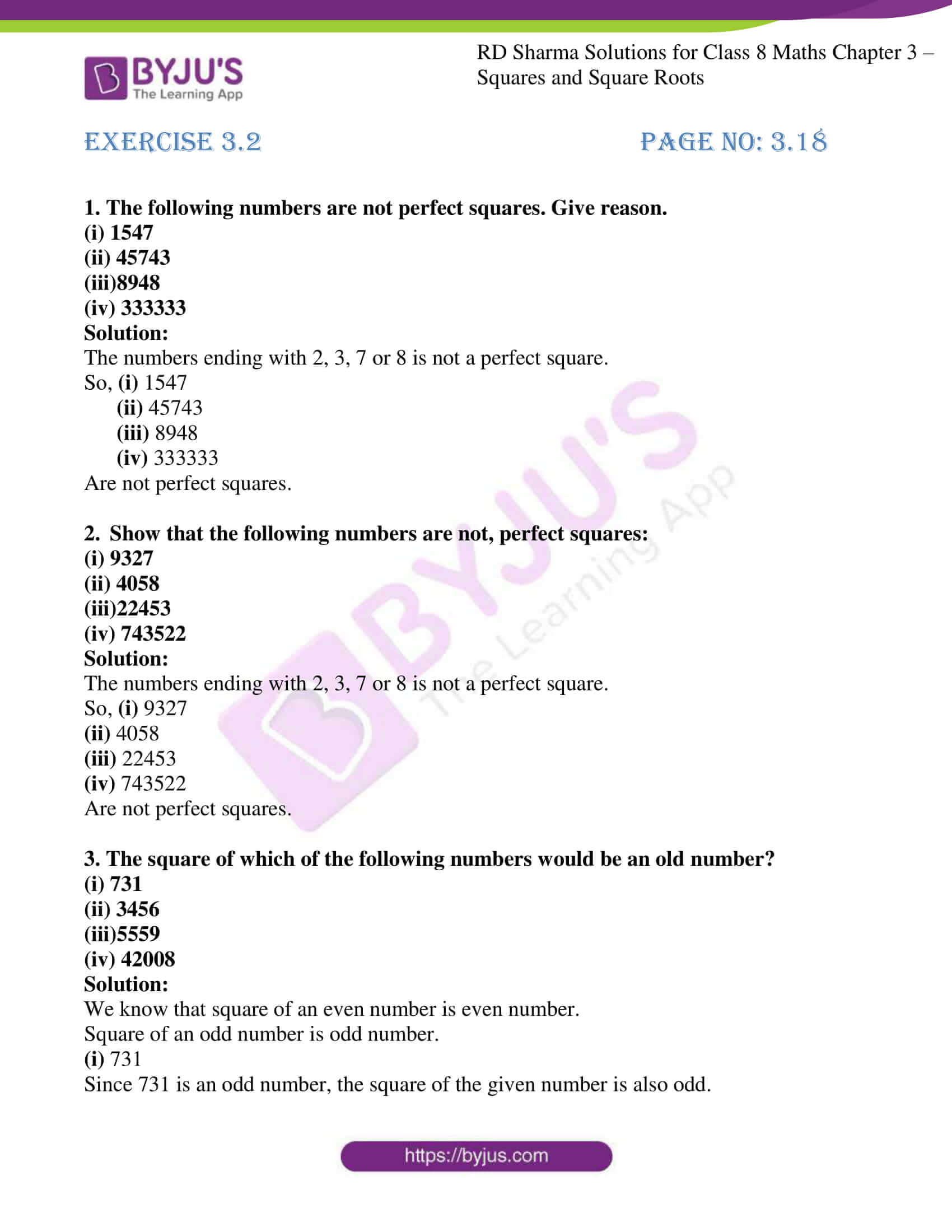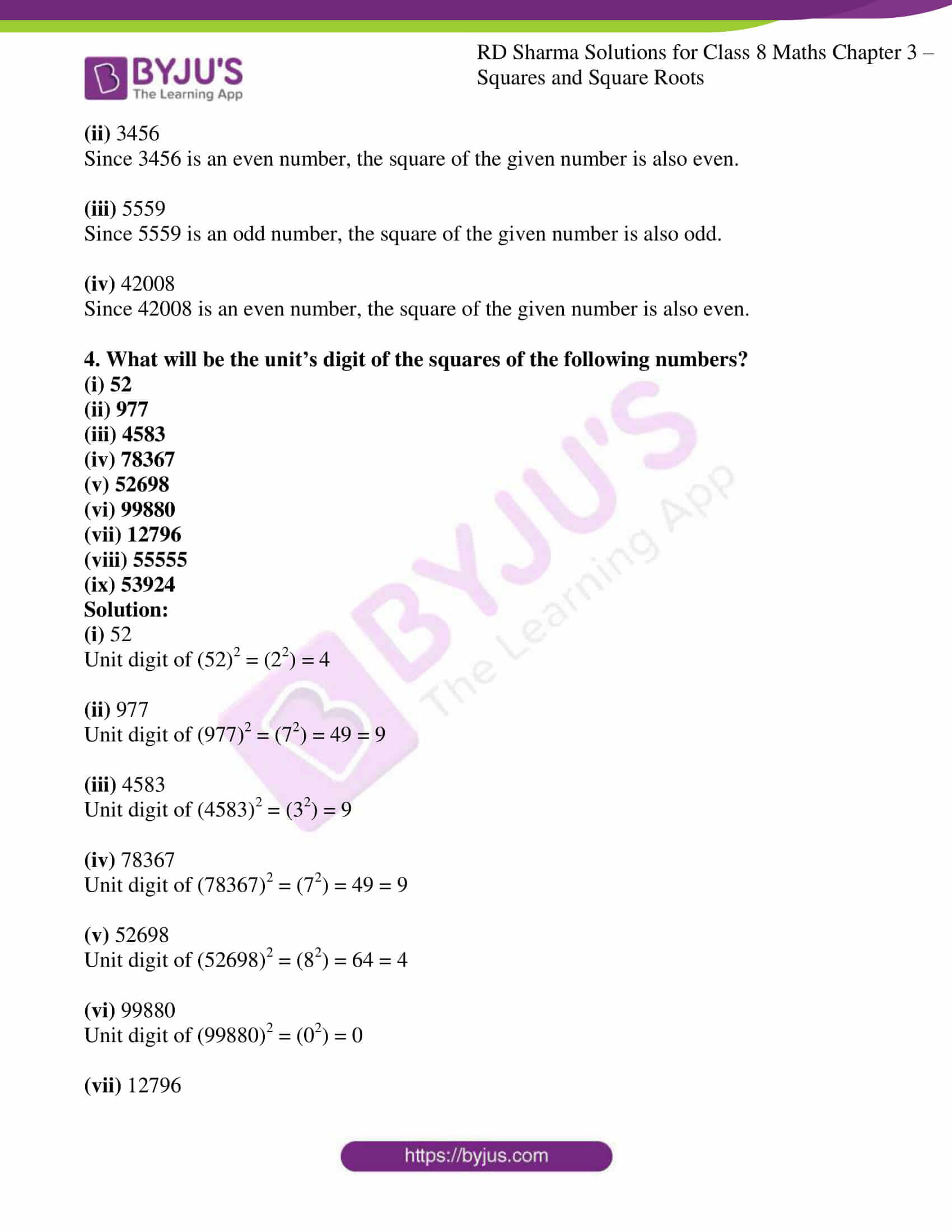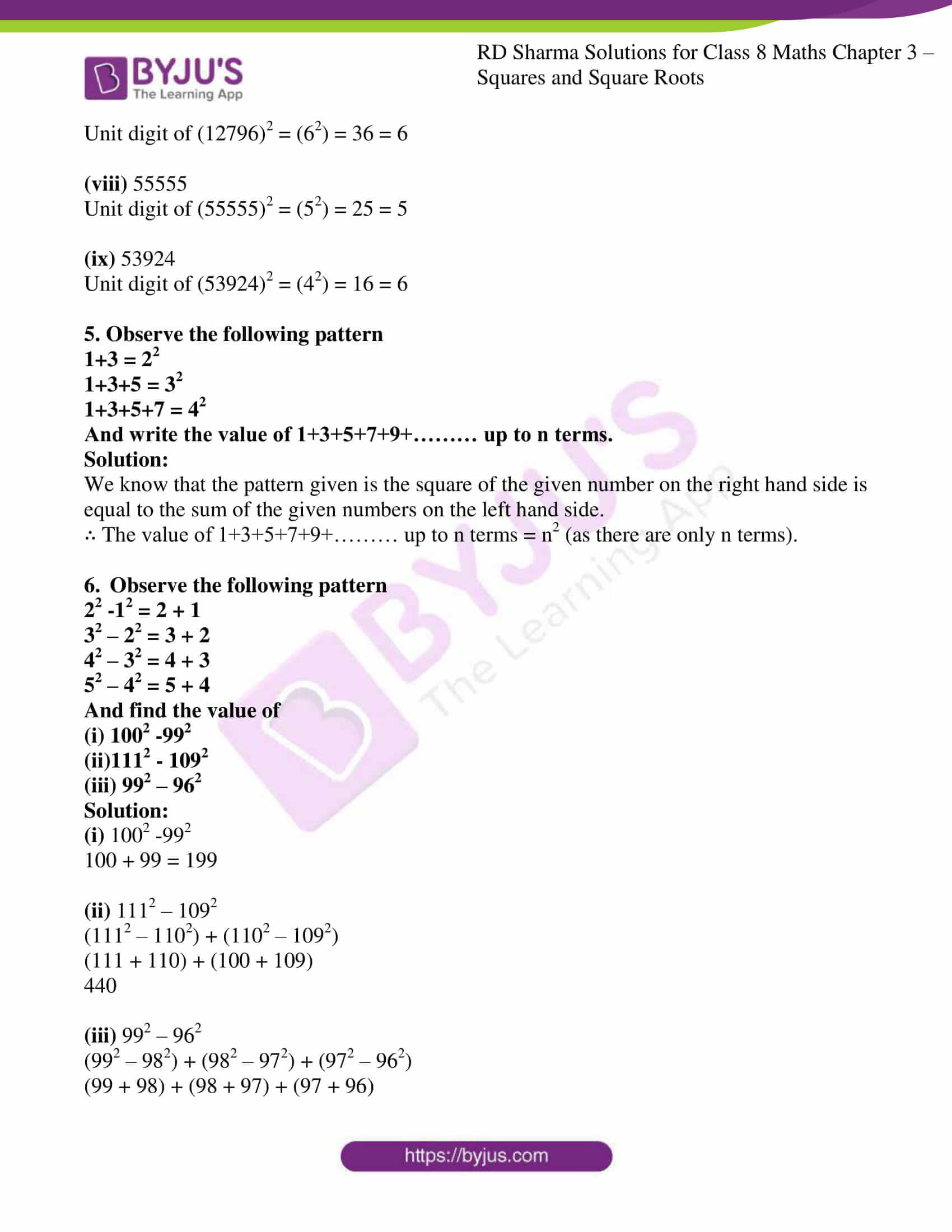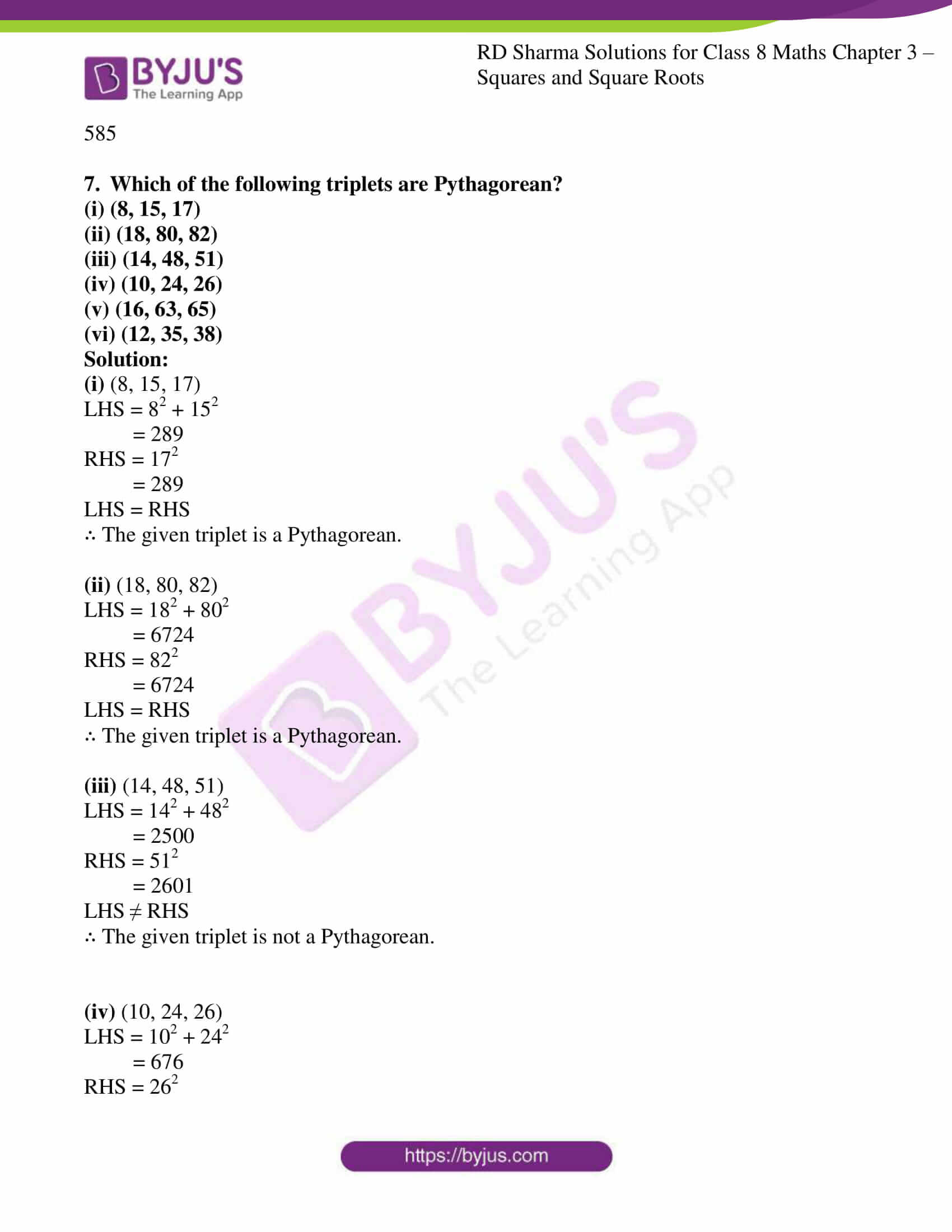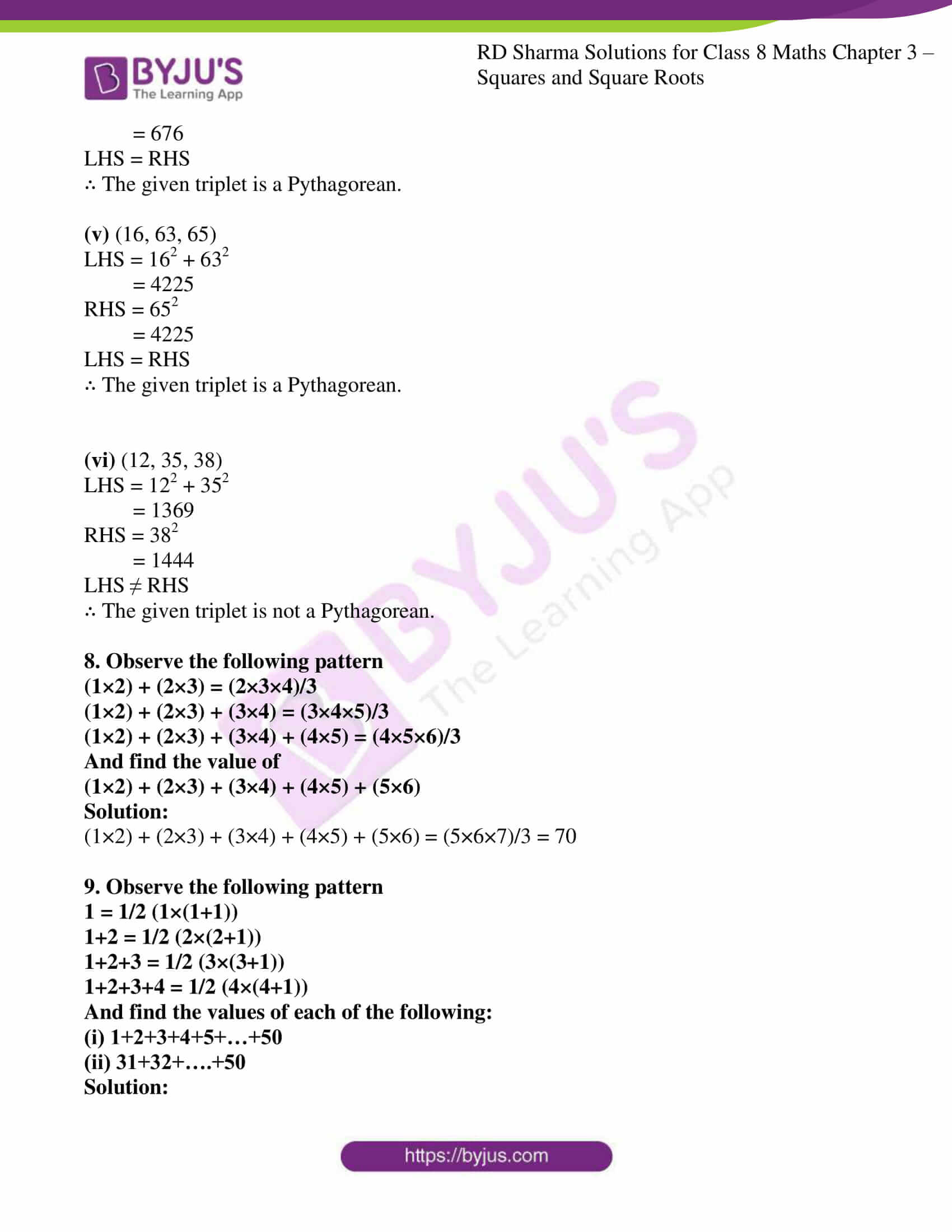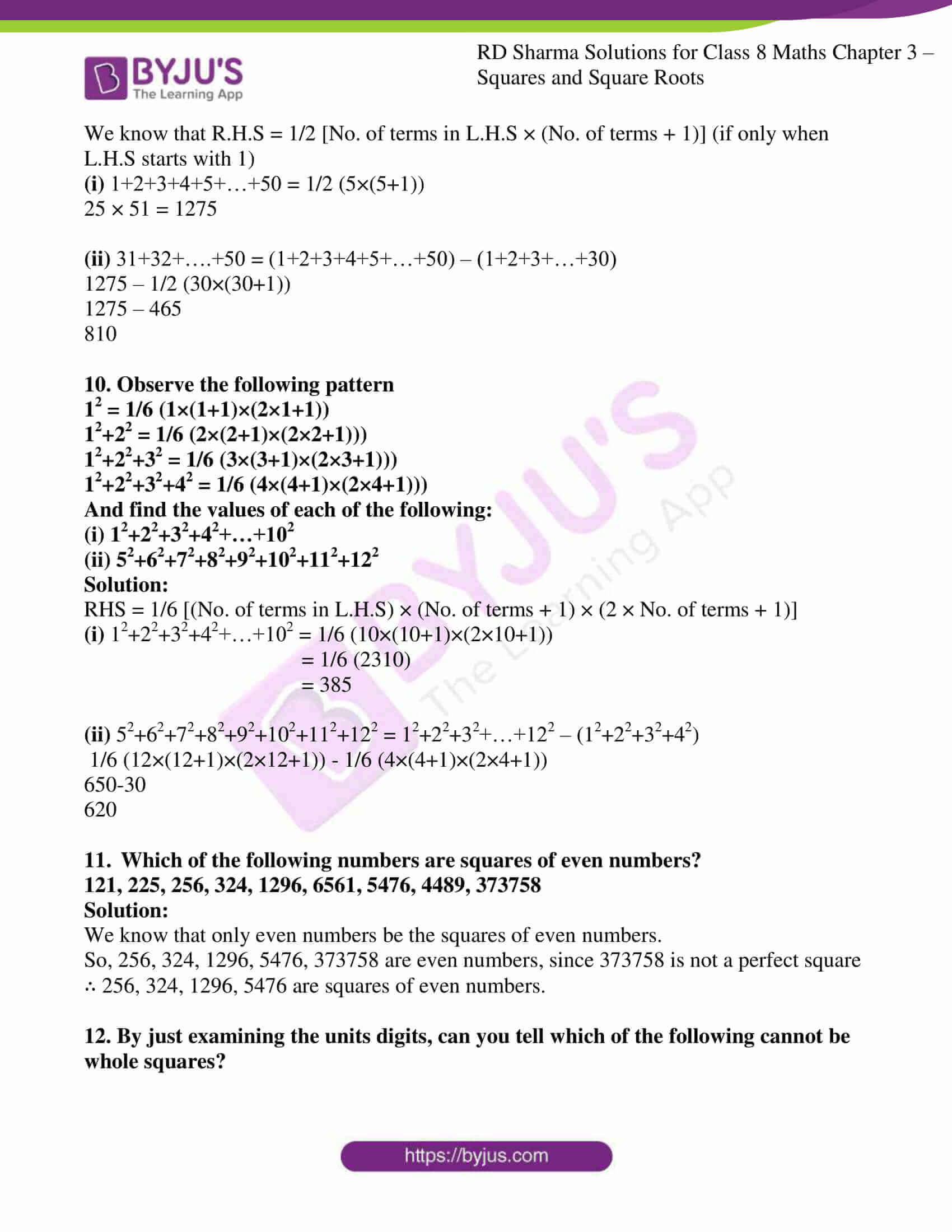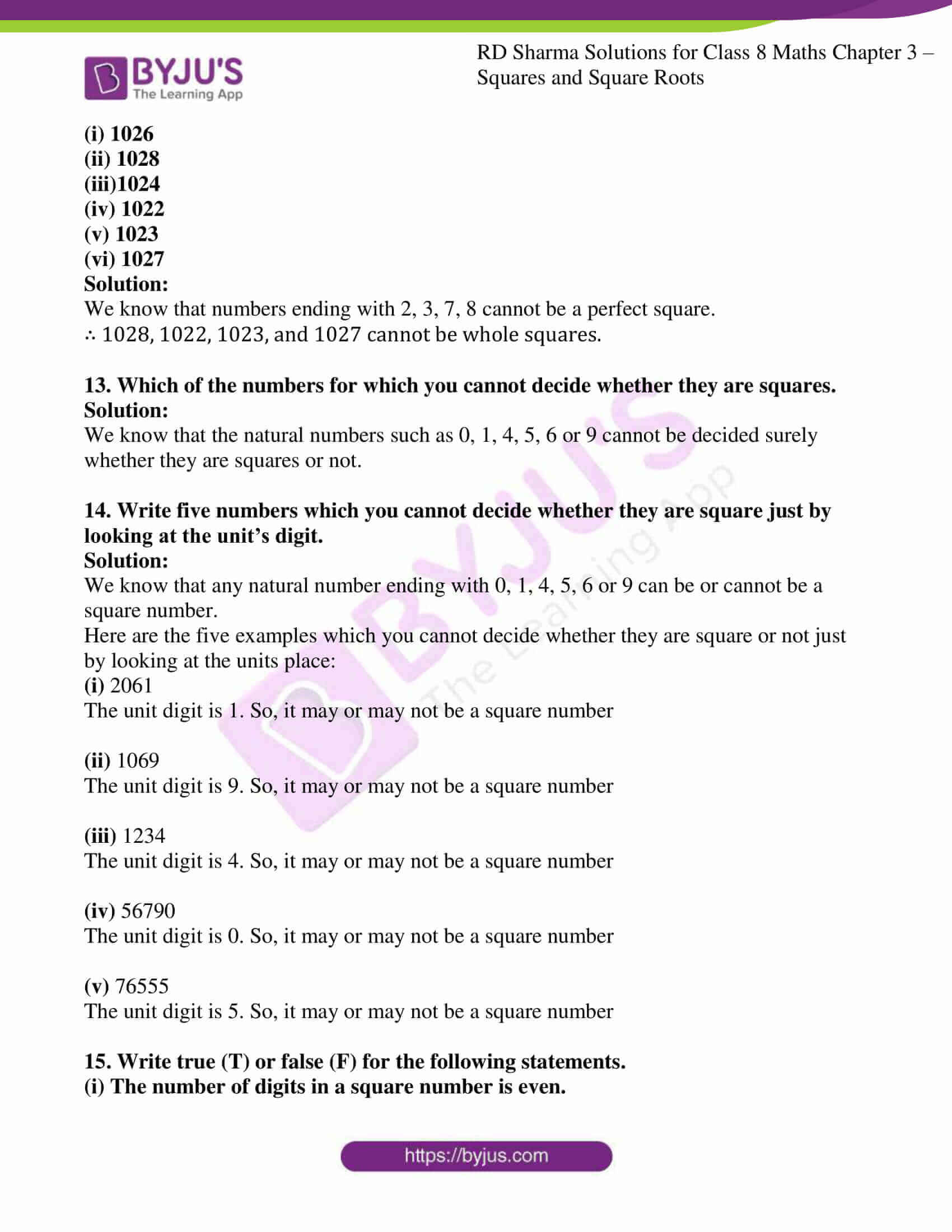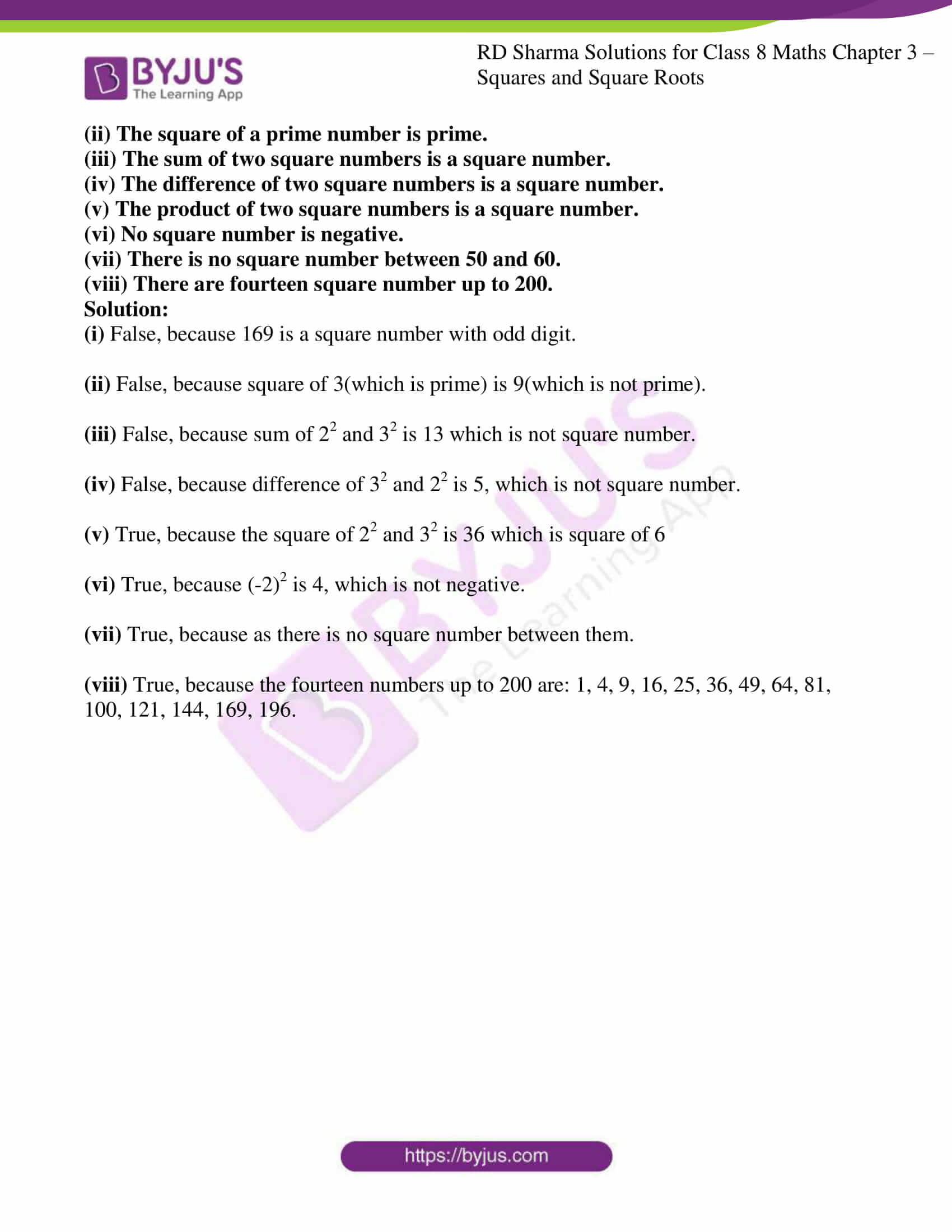### Access Answers to RD Sharma Solutions for Class 8 Maths Exercise 3.2 Chapter 3 Squares and Square Roots

#### EXERCISE 3.2 PAGE NO: 3.18

1. The following numbers are not perfect squares. Give reason.
(i) 1547

(ii) 45743
(iii)8948

(iv) 333333

Solution:

The numbers ending with 2, 3, 7 or 8 is not a perfect square.

So, (i) 1547

(ii) 45743
(iii)
8948

(iv) 333333

Are not perfect squares.

2. Show that the following numbers are not, perfect squares:
(i) 9327

(ii) 4058
(iii)22453

(iv) 743522

Solution:

The numbers ending with 2, 3, 7 or 8 is not a perfect square.

So, (i) 9327

(ii) 4058

(iii) 22453

(iv) 743522

Are not perfect squares.

3. The square of which of the following numbers would be an old number?
(i) 731

(ii) 3456
(iii)5559

(iv) 42008

Solution:

We know that square of an even number is even number.

Square of an odd number is odd number.

(i) 731

Since 731 is an odd number, the square of the given number is also odd.

(ii) 3456

Since 3456 is an even number, the square of the given number is also even.

(iii) 5559

Since 5559 is an odd number, the square of the given number is also odd.

(iv) 42008

Since 42008 is an even number, the square of the given number is also even.

4. What will be the unit’s digit of the squares of the following numbers?
(i) 52

(ii) 977
(iii) 4583

(iv) 78367
(v) 52698

(vi) 99880
(vii) 12796

(viii) 55555
(ix) 53924

Solution:

(i) 52

Unit digit of (52)2 = (22) = 4

(ii) 977

Unit digit of (977)2 = (72) = 49 = 9

(iii) 4583

Unit digit of (4583)2 = (32) = 9

(iv) 78367

Unit digit of (78367)2 = (72) = 49 = 9

(v) 52698

Unit digit of (52698)2 = (82) = 64 = 4

(vi) 99880

Unit digit of (99880)2 = (02) = 0

(vii) 12796

Unit digit of (12796)2 = (62) = 36 = 6

(viii) 55555

Unit digit of (55555)2 = (52) = 25 = 5

(ix) 53924

Unit digit of (53924)2 = (42) = 16 = 6

5. Observe the following pattern
1+3 = 22

1+3+5 = 32

1+3+5+7 = 42
And write the value of 1+3+5+7+9+……… up to n terms.

Solution:

We know that the pattern given is the square of the given number on the right hand side is equal to the sum of the given numbers on the left hand side.

∴ The value of 1+3+5+7+9+……… up to n terms = n2 (as there are only n terms).

6. Observe the following pattern

22 -12 = 2 + 1

32 – 22 = 3 + 2

42 – 32 = 4 + 3

52 – 42 = 5 + 4

And find the value of

(i) 1002 -992

(ii)1112 – 1092

(iii) 992 – 962

Solution:

(i) 1002 -992

100 + 99 = 199

(ii) 1112 – 1092

(1112 – 1102) + (1102 – 1092)

(111 + 110) + (100 + 109)

440

(iii) 992 – 962

(992 – 982) + (982 – 972) + (972 – 962)

(99 + 98) + (98 + 97) + (97 + 96)

585

7. Which of the following triplets are Pythagorean?
(i) (8, 15, 17)
(ii) (18, 80, 82)
(iii) (14, 48, 51)
(iv) (10, 24, 26)
(v) (16, 63, 65)
(vi) (12, 35, 38)

Solution:

(i) (8, 15, 17)

LHS = 82 + 152

= 289

RHS = 172

= 289

LHS = RHS

∴ The given triplet is a Pythagorean.

(ii) (18, 80, 82)

LHS = 182 + 802

= 6724

RHS = 822

= 6724

LHS = RHS

∴ The given triplet is a Pythagorean.

(iii) (14, 48, 51)

LHS = 142 + 482

= 2500

RHS = 512

= 2601

LHS ≠ RHS

∴ The given triplet is not a Pythagorean.

(iv) (10, 24, 26)

LHS = 102 + 242

= 676

RHS = 262

= 676

LHS = RHS

∴ The given triplet is a Pythagorean.

(v) (16, 63, 65)

LHS = 162 + 632

= 4225

RHS = 652

= 4225

LHS = RHS

∴ The given triplet is a Pythagorean.

(vi) (12, 35, 38)

LHS = 122 + 352

= 1369

RHS = 382

= 1444

LHS ≠ RHS

∴ The given triplet is not a Pythagorean.

8. Observe the following pattern

(1×2) + (2×3) = (2×3×4)/3

(1×2) + (2×3) + (3×4) = (3×4×5)/3

(1×2) + (2×3) + (3×4) + (4×5) = (4×5×6)/3

And find the value of

(1×2) + (2×3) + (3×4) + (4×5) + (5×6)

Solution:

(1×2) + (2×3) + (3×4) + (4×5) + (5×6) = (5×6×7)/3 = 70

9. Observe the following pattern

1 = 1/2 (1×(1+1))

1+2 = 1/2 (2×(2+1))

1+2+3 = 1/2 (3×(3+1))

1+2+3+4 = 1/2 (4×(4+1))

And find the values of each of the following:

(i) 1+2+3+4+5+…+50

(ii) 31+32+….+50

Solution:

We know that R.H.S = 1/2 [No. of terms in L.H.S × (No. of terms + 1)] (if only when L.H.S starts with 1)

(i) 1+2+3+4+5+…+50 = 1/2 (5×(5+1))

25 × 51 = 1275

(ii) 31+32+….+50 = (1+2+3+4+5+…+50) – (1+2+3+…+30)

1275 – 1/2 (30×(30+1))

1275 – 465

810

10. Observe the following pattern

12 = 1/6 (1×(1+1)×(2×1+1))

12+22 = 1/6 (2×(2+1)×(2×2+1)))

12+22+32 = 1/6 (3×(3+1)×(2×3+1)))

12+22+32+42 = 1/6 (4×(4+1)×(2×4+1)))

And find the values of each of the following:

(i) 12+22+32+42+…+102

(ii) 52+62+72+82+92+102+112+122

Solution:

RHS = 1/6 [(No. of terms in L.H.S) × (No. of terms + 1) × (2 × No. of terms + 1)]

(i) 12+22+32+42+…+102 = 1/6 (10×(10+1)×(2×10+1))

= 1/6 (2310)

= 385

(ii) 52+62+72+82+92+102+112+122 = 12+22+32+…+122 – (12+22+32+42)

1/6 (12×(12+1)×(2×12+1)) – 1/6 (4×(4+1)×(2×4+1))

650-30

620

11. Which of the following numbers are squares of even numbers?
121, 225, 256, 324, 1296, 6561, 5476, 4489, 373758

Solution:

We know that only even numbers be the squares of even numbers.

So, 256, 324, 1296, 5476, 373758 are even numbers, since 373758 is not a perfect square

∴ 256, 324, 1296, 5476 are squares of even numbers.

12. By just examining the units digits, can you tell which of the following cannot be whole squares?

(i) 1026

(ii) 1028
(iii)1024

(iv) 1022
(v) 1023

(vi) 1027

Solution:

We know that numbers ending with 2, 3, 7, 8 cannot be a perfect square.

∴ 1028, 1022, 1023, and 1027 cannot be whole squares.

13. Which of the numbers for which you cannot decide whether they are squares.

Solution:

We know that the natural numbers such as 0, 1, 4, 5, 6 or 9 cannot be decided surely whether they are squares or not.

14. Write five numbers which you cannot decide whether they are square just by looking at the unit’s digit.

Solution:

We know that any natural number ending with 0, 1, 4, 5, 6 or 9 can be or cannot be a square number.

Here are the five examples which you cannot decide whether they are square or not just by looking at the units place:

(i) 2061

The unit digit is 1. So, it may or may not be a square number

(ii) 1069

The unit digit is 9. So, it may or may not be a square number

(iii) 1234

The unit digit is 4. So, it may or may not be a square number

(iv) 56790

The unit digit is 0. So, it may or may not be a square number

(v) 76555

The unit digit is 5. So, it may or may not be a square number

15. Write true (T) or false (F) for the following statements.
(i) The number of digits in a square number is even.
(ii) The square of a prime number is prime.
(iii) The sum of two square numbers is a square number.
(iv) The difference of two square numbers is a square number.
(v) The product of two square numbers is a square number.
(vi) No square number is negative.
(vii) There is no square number between 50 and 60.
(viii) There are fourteen square number up to 200.

Solution:

(i) False, because 169 is a square number with odd digit.

(ii) False, because square of 3(which is prime) is 9(which is not prime).

(iii) False, because sum of 22 and 32 is 13 which is not square number.

(iv) False, because difference of 32 and 22 is 5, which is not square number.

(v) True, because the square of 22 and 32 is 36 which is square of 6

(vi) True, because (-2)2 is 4, which is not negative.

(vii) True, because as there is no square number between them.

(viii) True, because the fourteen numbers up to 200 are: 1, 4, 9, 16, 25, 36, 49, 64, 81, 100, 121, 144, 169, 196.

## RD Sharma Solutions for Class 8 Maths Exercise 3.2 Chapter 3 – Squares and Square Roots

Exercise 3.2 of RD Sharma Solutions for Chapter 3 Squares and Square Roots, deals with the basic concepts related to a perfect square. We can say that this exercise mainly deals with the properties of perfect squares such as Pythagorean Triplets, the sum of first ‘n’ odd natural numbers, etc. that the students have learned in this chapter.

The RD Sharma Solutions can help the students in practising and learning each and every concept as it provides solutions to all questions asked in the RD Sharma textbook. By practising the solutions students will be able to grasp the concepts perfectly. It also helps in boosting their confidence, which plays a crucial role in their examinations.